## Exercises

1. Define a semi-algebraic model that removes a triangular nose'' from the region shown in Figure 3.4.

2. For distinct values of yaw, pitch, and roll, it is possible to generate the same rotation. In other words,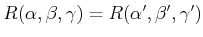for some cases in which at least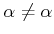,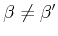, or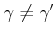. Characterize the sets of angles for which this occurs.

3. Using rotation matrices, prove that 2D rotation is commutative but 3D rotation is not.

4. An alternative to the yaw-pitch-roll formulation from Section 3.2.3 is considered here. Consider the following Euler angle representation of rotation (there are many other variants). The first rotation is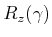, which is just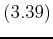with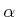replaced by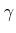. The next two rotations are identical to the yaw-pitch-roll formulation: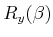is applied, followed by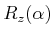. This yields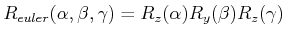.
1. Determine the matrix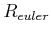.
2. Show that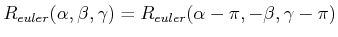.
3. Suppose that a rotation matrix is given as shown in (3.43). Show that the Euler angles are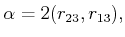(3.85)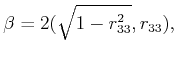(3.86)

and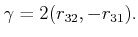(3.87)

5. There are 12 different variants of yaw-pitch-roll (or Euler angles), depending on which axes are used and the order of these axes. Determine all of the possibilities, using only notation such as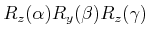for each one. Give brief arguments that support why or why not specific combinations of rotations are included in your list of 12.
6. Let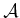be a unit disc, centered at the origin, and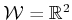. Assume thatis represented by a single, algebraic primitive,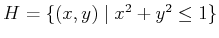. Show that the transformed primitive is unchanged after any rotation is applied.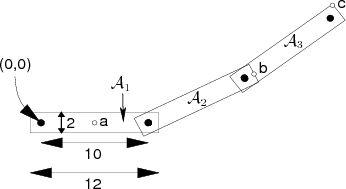7. Consider the articulated chain of bodies shown in Figure 3.29. There are three identical rectangular bars in the plane, called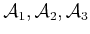. Each bar has width 2 and length 12. The distance between the two points of attachment is 10. The first bar,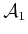, is attached to the origin. The second bar,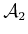, is attached to, and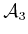is attached to. Each bar is allowed to rotate about its point of attachment. The configuration of the chain can be expressed with three angles,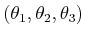. The first angle,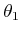, represents the angle between the segment drawn between the two points of attachment ofand the-axis. The second angle,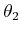, represents the angle betweenand(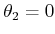when they are parallel). The third angle,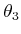, represents the angle betweenand. Suppose the configuration is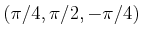.
1. Use the homogeneous transformation matrices to determine the locations of points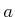,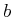, and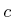.
2. Characterize the set of all configurations for which the final point of attachment (near the end of) is at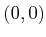(you should be able to figure this out without using the matrices).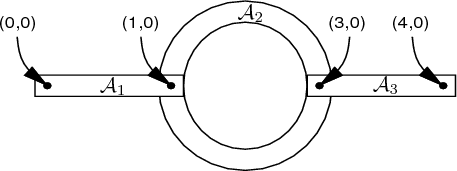8. A three-link chain of bodies that moves in a 2D world is shown Figure 3.30. The first link,, is attached atbut can rotate. Each remaining link is attached to another link with a revolute joint. The second link,, is a rigid ring, and the other two links are rectangular bars.

Assume that the structure is shown in the zero configuration. Suppose that the linkage is moved to the configuration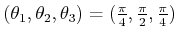, in whichis the angle of,is the angle ofwith respect to, andis the angle ofwith respect to. Using homogeneous transformation matrices, compute the position of the point at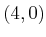in Figure 3.30, when the linkage is at configuration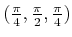(the point is attached to).

9. Approximate a spherical joint as a chain of three short, perpendicular links that are attached by revolute joints and give the sequence of transformation matrices. Show that as the link lengths approach zero, the resulting sequence of transformation matrices converges to exactly representing the freedom of a spherical joint. Compare this approach to directly using a full rotation matrix, (3.42), to represent the joint in the homogeneous transformation matrix.

10. Figure 3.12 showed six different ways in which 2D surfaces can slide with respect to each other to produce a joint.
1. Suppose that two bodies contact each other along a one-dimensional curve. Characterize as many different kinds of joints'' as possible, and indicate the degrees of freedom of each.
2. Suppose that the two bodies contact each other at a point. Indicate the types of rolling and sliding that are possible, and their corresponding degrees of freedom.

11. Suppose that two bodies form a screw joint in which the axis of the central axis of the screw aligns with the-axis of the first body. Determine an appropriate homogeneous transformation matrix to use in place of the DH matrix. Define the matrix with the screw radius,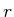, and displacement-per-revolution,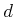, as parameters.

12. Recall Example 3.6. How should the transformations be modified so that the links are in the positions shown in Figure 3.25 at the zero configuration (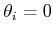for every revolute joint whose angle can be independently chosen)?

13. Generalize the shearing transformation of (3.84) to enable shearing of 3D models.

Implementations

14. Develop and implement a kinematic model for 2D linkages. Enable the user to display the arrangement of links in the plane.
15. Implement the kinematics of molecules that do not have loops and show them graphically as a ball and stick'' model. The user should be able to input the atomic radii, bond connections, bond lengths, and rotation ranges for each bond.
16. Design and implement a software system in which the user can interactively attach various links to make linkages that resemble those possible from using Tinkertoys (or another popular construction set that allows pieces to move). There are several rods of various lengths, which fit into holes in the center and around the edge of several coin-shaped pieces. Assume that all joints are revolute. The user should be allowed to change parameters and see the resulting positions of all of the links.
17. Construct a model of the human body as a tree of links in a 3D world. For simplicity, the geometric model may be limited to spheres and cylinders. Design and implement a system that displays the virtual human and allows the user to click on joints of the body to enable them to rotate.
18. Develop a simulator with 3D graphics for the Puma 560 model shown in Figure 3.4.

Steven M LaValle 2020-08-14